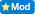-8+ x= -24

edited by moderator to add all the questions here....

2) = - 1/8 + y = - 3/4

3) x/5 = -6

4) 14- 6a = -2a + 3

0  Views: 606 Answers: 2 Posted: 11 years ago
ColleenPlease ask all your homework questions in one question. Do not post them separately. We typically do not do homework for students. There are some people who may tutor you if they are on however.

I guess by now, Laura Ann knows how to do these!

1.)   -8 + x = -24           >           -8 + x = -24

+8           +8

x  =  -16

2.)   -1/8 + y = -3/4        >           -1/8 + y = -3/4

8(-1/8 + y) = 8(-3/4)

-1 + 8y  =  -6

+1             +1

8y  =  -5

8         8

y  =  -5/8

3.)    x/5  =  -6                   >             x/5  =  -6

5(x/5)  =  5(-6)

x    =  -30

4.)     14 - 6a = -2a + 3         >          14 - 6a  =  -2a + 3

-14 +2a  =  +2a -14

-4a   =   -11

-4          -4

a  =  11/4

What is 24-8?? find that make it a negative # and you'll have answer #1

For Q #2 start by finding the common denominator 1/8 and 3/4 once you have done that finding y won't be so hard

For more help try https://www.algebrahelp.com

### Top contributors in Uncategorized category

ROMOS
Karma: 1101K

Colleen
Karma: 953K

country bumpkin
Karma: 838K

Benthere
Karma: 760K
> Top contributors chart

massage centre in mysore
Answers: 0 Views: 6 Rating: 0
Monitor: output: memory card
Answers: 0 Views: 8 Rating: 0
> More questions...

449673
questions
718592